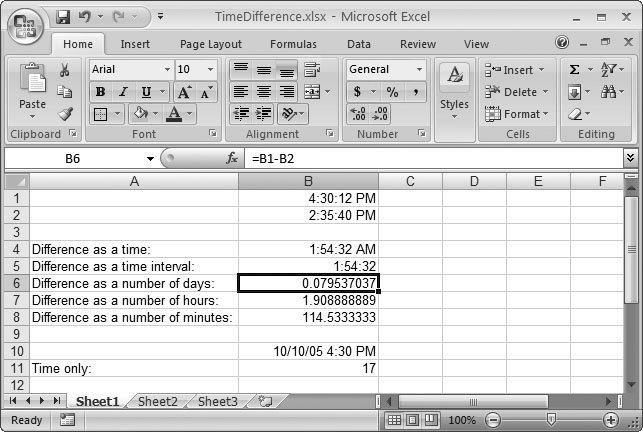# Section 11.3. Math with Dates and Times

### 11.3. Math with Dates and Times

On Planet Excel, since dates and times are really just special types of numbers , you can use them in calculations like addition, subtraction, and so on. In the case of dates, the most common operation is to subtract one date from another to calculate the number of days in between. For example, consider this formula:

` =A2-A1 `

If A2 contains the value 10/30/2007, and A1 contains the value 3/20/2007, the result is 224, which is the number of days between these two dates.

#### 11.3.1. Using Dates and Times with Ordinary Functions

Of course, you can also use other Excel functions in your calculations. For example, you could take a number that represents a combined date and time value, extract just the fractional time portion, and round it to a number of whole hours using the following formula:

` =ROUND((A1-INT(A1))*24, 0) `

This formula works on a date in cell A1. Here's how it breaks down. The formula begins by calculating INT(A1) . This function truncates the date number, removing the fractional time portion. Then, the formula evaluates A1-INT(A1) . Now you've got just the fractional time portion of the date. Next , multiply this fractional part by 24 (the number of hours in a day), and then round it to a whole number. The end result is that you extract the number of hours in a date/time value. Figure 11-3 shows some examples of date calculations similar to this one.Figure 11-3. This worksheet shows different ways to find the difference between two times.

In Figure 11-3, the calculation shown in cell B4 ( =B1-B2 ) uses the default format, which incorrectly interprets the time difference as a chronological time value. The second calculation (cell B5) uses the same formula but an interval time format. As a result, Excel displays the result as an interval of time. The next example (cell B6) uses the General format to display the result in terms of the number of fractional days. Finally, the last two examples take the additional step of multiplying the number of fractional days by 24 (to calculate fractional hours) and by 60 (to calculate fractional minutes).

As you'll see in the following sections, Excel has plenty more specialized date functions that often make calculations easier than this.

Note: Remember, if you change the number format, you may end up hiding part of the date's information. For example, if you choose to display a combined date and time value using a time-only format, you won't see the date information. However, Excel still uses this date information in any calculations you perform, which could lead to unexpected data. For best results, perform your date manipulation using the date functions described in the next chapter, rather than writing your own formulas from scratch.Excel 2007[c] The Missing Manual
ISBN: 596527594
EAN: N/A
Year: 2007
Pages: 173